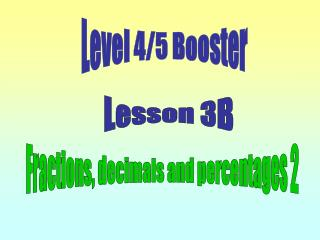DownloadDownload PresentationLevel 4/5 Booster

# Level 4/5 Booster

Download Presentation## Level 4/5 Booster

- - - - - - - - - - - - - - - - - - - - - - - - - - - E N D - - - - - - - - - - - - - - - - - - - - - - - - - - -
##### Presentation Transcript

1. Level 4/5 Booster Lesson 3B Fractions, decimals and percentages 2

2. Objectives: • Calculate percentages using non-calculator methods. • Calculate percentages less than 10% and values other than multiples of 10. Vocabulary: multiple Equivalent recurring decimal

3. T3.1B 500 is 10% of 5000 20 is 50% of 40. 50 is 20% of 250 120 is 25% of 480 90 is 5% of 1800 70 is 50% of 140 60 is 50% of 120 120 is 40% of 300 250 is 50% of 500 90 is 75% of 120 Find three more percentage relationships on the target board.

4. What is 10% of £380? How did you work out 10% of £380? How can you use the answer for 10% to find 20%? 20% = 10% + 10% = £38 + £38 = £76 How can you use 10% to find 30%? 30% = 10% + 10% + 10% = 3 x £38 = £114

5. W/S 3.1B 10% 60% 50% 20% 60 30% 40% On a copy of the spider diagram fill in the amounts.

6. Answers: 10% 60% 6 36 50% 20% 60 30 12 30% 40% 24 18

7. How many times will 5% fit into 10%? How can you work out 5% if you know 10%? Find 5% of £240 – explain how you got your answer. 10% of £240 is £24 so 5% of £240 is £12. 10% 0% 100% 0 £240 £24 Find 5% of £720 – explain how you got the answer. so 5% of £720 is £36 10% of £720 is £72

8. T3.2B Find 5% of each amount as it is highlighted

9. How can you find 15% of an amount? 15% can be found by adding 10% and 5% together. Find 15% of 300 – explain your working. 10% of 300 is 30 5% of 300 is 15 15% is 30 + 15 = 45 15% 10% 0% 100% 0 300 30 45

10. T3.2B Find 15% of each number as it is highlighted.

11. W/S3.2B 25% 15% 10% 17.5% 5% 60 45% 95% 35% 55% Complete a spider diagram like the one above.

12. Answers 25% 15% 10% 9 15 6 17.5% 10.5 5% 3 60 45% 57 95% 27 21 33 35% 55%

13. If I can find 10% how can I find 1%? Example: Find 1% of £350 Method 1 1% is the same as 1/100th so I can divide by 100 £350 ÷ 100 = £3.5 written £3.50 Method 2 1% is 10% divided by 10. 10% of £350 is £35 so 1% is £35 ÷10 = £3.5 or £3.50

14. T3.1B State 1% of each number as it is highlighted.

15. Objectives: • Calculate percentages using non-calculator methods. • Calculate percentages less than 10% and values other than multiples of 10. Vocabulary: multiple Equivalent recurring decimal

16. Thank you for your attention